# Recognizing Handwritten Digits with scikit-learn

Recognizing handwritten text is a problem that can be traced back to the first automatic machines that needed to recognize individual characters in the handwritten documents.

Think about, for example, the ZIP codes on letters at the post office and the automation needed to recognize these five digits. Perfect recognition of these codes is necessary in order to sort mail automatically and efficiently.

The Hypothesis to be tested :

“The Digits data set of scikit-learn library provides numerous data-sets that are useful for testing many problems of data analysis and prediction of the results. Some Scientist claims that it predicts the digit accurately 95% of the times. Perform data Analysis to accept or reject this Hypothesis.”

This article presents recognizing the handwritten digits (0 to 9) using the famous digits data set from Scikit-Learn, using three different algorithms.

1. Logistic Regression Classifier
2. Support Vector Machine Classifier
3. KNN ClassifierHandwritten Digits

Digits data set consists of 1,797 images that are 8x8 pixels in size. Each image is a handwritten digit in grayscale.

Scikit-Learn is a library for Python that contains numerous useful algorithms that can easily be implemented and altered for the purpose of classification and other machine learning tasks.

One of the most fascinating things about the Scikit-Learn library is that is has a 4-step modeling pattern that makes it easy to code a machine learning classifier:

Import the model you want to use.

Make an instance of the Model.

Training the model on the data and storing the information learned from the data.

Predicting the labels of new data

# Implementation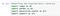Importing the required basic libraries

The Scikit-learn library provides numerous datasets, among which we will be using a data set of images called Digits. This data set consists of 1,797 images that are 8x8 pixels in size. Each image is a handwritten digit in grayscale.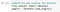Importing and loading the dataset

Information about any specific dataset can be obtained by calling the DESCR attribute on that dataset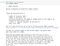Description of the dataset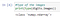Checking the datatype of the images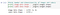Total number of images and labels

The images of the handwritten digits are contained in a digits.images array. Each element of this array is an image that is represented by an 8x8 matrix of numerical values that correspond to a grayscale from white, with a value of 0, to black, with the value 15.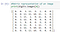Matrix representation of an image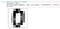Visual representation of an image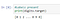Values of the labels present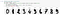Visualizing the first ten images and labels in the Dataset

Now let’s split our Dataset into training and validation sets to make sure that after we train our model, it is able to generalize well to new data.

# Scikit-Learn 4-Step Modeling Pattern

Here, we have used three different algorithms for the recognition of the handwritten digits and also measured the accuracy obtained from all the algorithms

1. Logistic Regression Classifier

Here we will be using Logistic Regression. Logistic regression is a linear classifier and therefore used when there is some sort of linear relationship between the data.Importing the model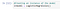Making an instance of the Model

Here the Model is learning the relationship between digits (X_train) and labels (Y_train)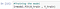Training the Model

Using the information the Model learned during the training process.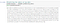Predicting the labels of new data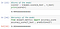Performance of the model

This number is the probability for the digits in the test sample to be classified in the right category, meaning that we get 96.94% of the digits correct.

Thus this model is almost ~97% accurate in recognizing the handwritten digits

A confusion matrix is a table that is often used to evaluate the accuracy of a classification model. We will be using Seaborn library for our confusion matrix.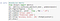Using Seaborn library to plot the confusion matrix

The confusion matrix is as follows: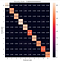Confusion Matrix

2. Support Vector Machine Classifier

Here we will be using Support Vector Machine Classifier. A support vector machine better known as SVM is a supervised machine learning model that uses classification algorithms for two-group classification problems. After giving an SVM model sets of labeled training data for each category, they’re able to categorize new text.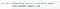Importing the model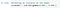Making an instance of the Model

Here the Model is learning the relationship between digits (X_train) and labels (Y_train)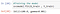Training the Model

Using the information the Model learned during the training process.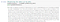Predicting the labels of new data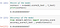Performance of the model

This number is the probability for the digits in the test sample to be classified in the right category, meaning that we get 99.17% of the digits correct.

Thus this model is 99.17% accurate in recognizing the handwritten digits

A confusion matrix is a table that is often used to evaluate the accuracy of a classification model. We will be using Seaborn library for our confusion matrix.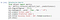Using Seaborn library to plot the confusion matrix

The confusion matrix is as follows: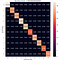Confusion Matrix

3. KNN Classifier

Here we will be using KNN Classifier. K-Nearest Neighbors (KNN) is one of the simplest algorithms in Machine Learning for regression and classification problem. KNN algorithms use data and classify new data points based on similarity measures (e.g. distance function). Classification is done by a majority vote to its neighbors.Importing the model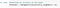Making an instance of the Model

Here the Model is learning the relationship between digits (X_train) and labels (Y_train)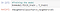Training the Model

Using the information the Model learned during the training process.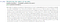Predicting the labels of new data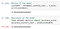Performance of the model

This number is the probability for the digits in the test sample to be classified in the right category, meaning that we get 99.44% of the digits correct.

Thus this model is 99.44% accurate in recognizing the handwritten digits

A confusion matrix is a table that is often used to evaluate the accuracy of a classification model. We will be using Seaborn library for our confusion matrix.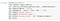Using Seaborn library to plot the confusion matrix

The confusion matrix is as follows: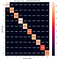Confusion Matrix

Thus we can conclude that the hypothesis given is accepted for all the three algorithms (Logistic Regression , SVM Classifier , KNN Classifier). The accuracy of predictions obtained from all the three algorithms is greater than 95% (Logistic Regression ~ 96.95% , SVM Classifier ~ 99.17% , KNN Classifier ~ 99.44%). From this article, one can perform all the necessary steps to import a dataset, build a model using Scikit-Learn, train the model, make predictions with it, and find the accuracy of the prediction. Hence by using Scikit-Learn library in python,data analysis becomes easy ,effective and take less time.